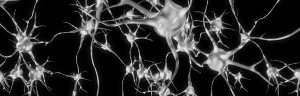# Month: February 2014

## Neural Networks, Part 3: The NetworkWe have learned about individual neurons in the previous section, now it’s time to put them together to form an actual neural network.

The idea is quite simple – we line multiple neurons up to form a layer, and connect the output of the first layer to the input of the next layer. Here is an illustration:

We have 3 features (vector space dimensions) in the input layer that we use for learning: $$x_1$$, $$x_2$$ and $$x_3$$. The first hidden layer has 3 neurons, the second one has 2 neurons, and the output layer has 2 output values. The size of these layers is up to you – on complex real-world problems we would use hundreds or thousands of neurons in each layer.